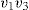Chapter 30

Triangulating the Interior of a Convex Polygon for the Second Time

Now it is time to revisit the situation we examined in Example 23.1.

Example 30.1: In this example, we shall take a second look at the problem of determining Tn, the number of ways the interior of a convex polygon of n sides can be triangulated into n − 2 triangles—by drawing n − 3 diagonals, no two of which intersect within the interior.

In Fig. 30.1 30.1 (a), we have a convex polygon with n + 1 sides, where we have drawn the diagonalsand. These diagonals break up the interior of this convex polygon into three regions: (i) the interior of triangle; (ii) the interior of triangle; and (iii) the interior of the (n − 1) -sided convex polygon. The interior of the first region can be triangulated in T3ways, while there are Tn−1 = T(n+1)−2 ways to triangulate the interior of the third region. Therefore, there are T3T(n+1)−2 ways to triangulate the interior of the given convex polygon with n + 1 sides—where the ...

Get Fibonacci and Catalan Numbers: An Introduction now with the O’Reilly learning platform.

O’Reilly members experience live online training, plus books, videos, and digital content from nearly 200 publishers.